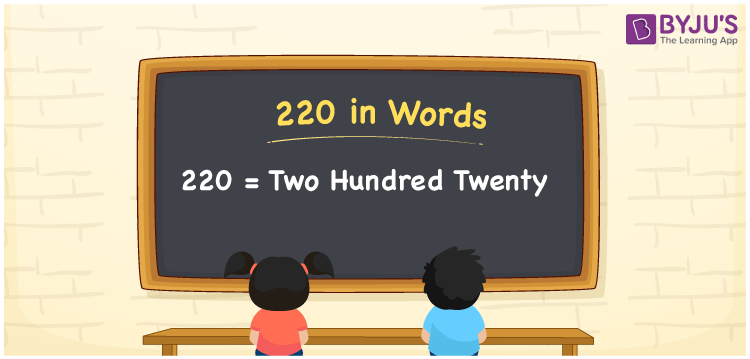# 220 in Words

220 in words can be written as Two Hundred Twenty. The concepts like counting numbers which are important from primary education are discussed here in brief. For instance, if you spend Rs. 220 in buying a cap, then you can say that “I bought a cap for Two Hundred Twenty Rupees”. The English alphabet is used to write 220 in words. Students can get a clear knowledge of numbers in words with the help of the study materials given at BYJU’S. The number 220 can be read as “Two Hundred Twenty” in English.

 220 in words Two Hundred Twenty Two Hundred Twenty in Numbers 220

## 220 in English Words## How to Write 220 in Words?

Place value chart and expanded form of 220 can be learn easily in this section. There are three digits in the number 220. The place value chart for 220 is provided below for reference to help students understand the concepts effectively.

 Hundreds Tens Ones 2 2 0

The expanded form of 220 is provided below:

2 × Hundred + 2 × Ten + 0 × One

= 2 × 100 + 2 × 10 + 0 × 1

= 220

= Two Hundred Twenty

Hence, 220 in words is written as Two Hundred Twenty.

220 is a natural number that precedes 221 and succeeds 219.

220 in words – Two Hundred Twenty

Is 220 an odd number? – No

Is 220 an even number? – Yes

Is 220 a perfect square number? – No

Is 220 a perfect cube number? – No

Is 220 a prime number? – No

Is 220 a composite number? – Yes

## Frequently Asked Questions on 220 in Words

Q1

### How can 220 be written in words?

220 can be written as “Two Hundred Twenty” in words.
Q2

### Is 220 an odd number?

No, 220 is not an odd number because it is divisible by 2.
220/2 = 110
Q3

### Write Two Hundred Twenty in numbers.

Two Hundred Twenty can be written in numbers as 220.